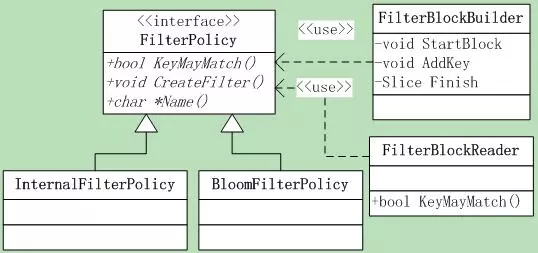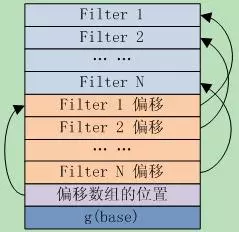• 2021-01-11
• 浏览 (436)

# leveldb源码分析12

## 8.FilterPolicy&Bloom之1

### 8.1 FilterPolicy

``````virtual const char* Name() const = 0;
// 返回filter的名字
virtual void CreateFilter(const Slice* keys,
int n, std::string* dst)const = 0;
virtual bool KeyMayMatch(const Slice& key, const Slice& filter)const = 0;
``````

CreateFilter接口，它根据指定的参数创建过滤器，并将结果append到dst中，注意：不能修改dst的原始内容，只做append。 参数@keys[0,n-1]包含依据用户提供的comparator排序的key列表–可重复，并把根据这些key创建的filter追加到@*dst中。 KeyMayMatch，参数@filter包含了调用CreateFilter函数append的数据，如果key在传递函数CreateFilter的key列表中，则必须返回true### 8.2InternalFilterPolicy

``````constFilterPolicy* const user_policy_;
``````

Name()返回的是userpolicy->Name()

``````bool InternalFilterPolicy::KeyMayMatch(const Slice& key, constSlice& f) const
{
returnuser_policy_->KeyMayMatch(ExtractUserKey(key), f);
}
void InternalFilterPolicy::CreateFilter(const Slice* keys,
int n,std::string* dst) const
{
Slice* mkey =const_cast<Slice*>(keys);
for (int i = 0; i < n; i++)mkey[i] = ExtractUserKey(keys[i]);
user_policy_->CreateFilter(keys, n, dst);
}
``````

### 8.3 BloomFilter

#### 8.3.1 基本理论

Bloom Filter实际上是一种hash算法，数学之美系列有专门介绍。它是由巴顿.布隆于一九七零年提出的，它实际上是一个很长的二进制向量和一系列随机映射函数。 Bloom Filter将元素映射到一个长度为m的bit向量上的一个bit，当这个bit是1时，就表示这个元素在集合内。使用hash的缺点就是元素很多时可能有冲突，为了减少误判，就使用k个hash函数计算出k个bit，只要有一个bit为0，就说明元素肯定不在集合内。下面的图8.3-1是一个示意图。leveldb的实现中，Name()返回”leveldb.BuiltinBloomFilter”，因此metaindex block** 中的key就是”filter.leveldb.BuiltinBloomFilter”。Leveldb使用了double hashing来模拟多个hash函数，当然这里不是用来解决冲突的。 和线性再探测（linearprobing）一样，Double hashing从一个hash值开始，重复向前迭代，直到解决冲突或者搜索完hash表。不同的是，double hashing使用的是另外一个hash函数，而不是固定的步长。 给定两个独立的hash函数h1和h2，对于hash表T和值k，第i次迭代计算出的位置就是：h(i, k) = (h1(k) + i*h2(k)) mod |T|。 对此，Leveldb选择的hash函数是：

``````Gi(x)=H1(x)+iH2(x)
H2(x)=(H1(x)>>17) | (H1(x)<<15)
``````

H1是一个基本的hash函数，H2是由H1循环右移得到的，Gi(x)就是第i次循环得到的hash值。【理论分析可参考论文Kirsch,Mitzenmacher2006】 在bloom_filter的数据的最后一个字节存放的是k_的值，k_实际上就是G(x)的个数，也就是计算时采用的hash函数个数

#### 8.3.2 BloomFilter参数

``````bits_per_key_ = bits_per_key;
k_ =static_cast<size_t>(bits_per_key * 0.69); // 0.69 =~ ln(2)
if (k_ < 1) k_ = 1;
if (k_ > 30) k_ = 30;
``````

#### 8.3.3 建立BloomFilter

``````void CreateFilter(const Slice* keys, int n, std::string* dst) const
``````

##### S1 首先根据key个数分配filter空间，并圆整到8byte。
``````size_t bits = n * bits_per_key_;
if (bits < 64) bits = 64;
// 如果n太小FP会很高，限定filter的最小长度
size_t bytes = (bits + 7) / 8;    // 圆整到8byte
bits = bytes * 8;                 // bit计算的空间大小
const size_t init_size =dst->size();
dst->resize(init_size +bytes, 0); // 分配空间
``````
##### S2 在filter最后的字节位压入hash函数个数
``````dst->push_back(static_cast<char>(k_));
// Remember # of probes in filter
``````
##### S3 对于每个key，使用double-hashing生产一系列的hash值h(K_个)，设置bits array的第h位=1。
``````char* array =&(*dst)[init_size];
for (size_t i = 0; i < n;i++)
{
// double-hashing，分析参见[Kirsch,Mitzenmacher 2006]
uint32_t h =BloomHash(keys[i]);
// h1函数
const uint32_t delta = (h>> 17) | (h << 15);
// h2函数、由h1 Rotate right 17 bits
for (size_t j = 0; j <k_; j++)
{
// double-hashing生产k_个的hash值
const uint32_t bitpos = h% bits;
// 在bits array上设置第bitpos位
array[bitpos/8] |= (1<< (bitpos % 8));
h += delta;
}
}
``````

Bloom Filter的创建就完成了。

#### 8.3.4 查找BloomFilter

##### S1 准备工作，并做些基本判断。
``````
const size_t len =bloom_filter.size();
if (len < 2) return false;
const char* array = bloom_filter.data();
const size_t bits = (len - 1)* 8;
const size_t k = array[len-1];
// 使用filter的k，而不是k_，这样更灵活
if (k > 30) return true;
// 为短bloom filter保留，当前认为直接match
``````
##### S2 计算key的hash值，重复计算阶段的步骤，循环计算k个hash值，只要有一个结果对应的bit位为0，就认为不匹配，否则认为匹配。
``````uint32_t h = BloomHash(key);
const uint32_t delta = (h>> 17) | (h << 15);  // Rotate right 17 bits
for (size_t j = 0; j < k;j++)
{
const uint32_t bitpos = h %bits;
if ((array[bitpos/8] &(1 << (bitpos % 8))) == 0) return false;
// notmatch
h += delta;
}
return true; // match
``````

### 8.4 Filter Block格式

Filter Block也就是前面sstable中的meta block，位于data block之后。

Filter block存储了一连串的filter值，其中第i个filter保存的是block b中所有的key通过FilterPolicy::CreateFilter()计算得到的结果，block b在sstable文件中的偏移满足[ i*base … (i+1)*base-1 ]